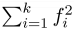## Day 2, Problem 3: Repetitivity

Any string of length n has 2n subsequences, which are the strings obtained by deleting some subset of the characters. But these subsequences may not all be distinct. For example, the string zoo has only 6 distinct subsequences:

• the subsequences z, oo, and zoo appear only once,
• the empty subsequence appears only once,
• and the subsequences o and zo each appear twice.

Suppose a string S has k distinct subsequences, and that the ith one appears fi times. Then the repetitivity of S is defined as$\sum_{i=1}^{k} f_{i}^{2}$. For example, the repetitivity of zoo is

12 + 12 + 12 + 12 + 22 + 22 = 12.

### Input

Each test case contains a line containing the string S (with length at most 10000), followed by a line containing a single integer M (2 ≤ M ≤ 109). You may assume that S only contains characters with ASCII codes between 33 and 126 inclusive (these are all printable, nonwhitespace characters).

For test cases worth 20% of the points, you may assume that S will be at most 20 characters long.

### Output

The output should consist of a single line, containing the repetitivity of S, modulo M.

### Sample Input 1

zoo
10

### Sample Output 1

2

### Sample Input 2

@#\$%
1000000

### Sample Output 2

16

Point Value: 25 (partial)
Time Limit: 10.00s
Memory Limit: 512M